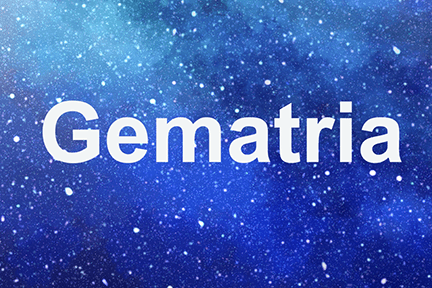# Gematria

There are different systems of interpretation of the hidden meanings of the Torah. One of them is the Gematria, where the mathematical values of each letter or word are calculated. Each letter having its own numerical value, the fact that some words have the same total is not just coincidence, but denotes a similarity or complementarity.

It is one of the different systems of interpretation of the hidden meanings of the Torah, where mathematical values of letters, words, and sentences are calculated to find a similarity or complementarity. Each letter has its own numerical value.

 Letter Name Value א Aleph 1 ב Beit 2 ג Gimel 3 ד Dalet 4 ה He 5 ו Vav 6 ז Zain 7 ח ‘het 8 ט Tet 9 י Yud 10 כ Khaf 20 ל Lamed 30 מ Mem 40 נ Nun 50 ס Samekh 60 ע ‘ain 70 פ Pey 80 צ Tsadey 90 ק Kuf 100 ר Resh 200 ש Shin 300 ת Tav 400

The final letters also have their own numerical values:

 Letter Name Value ך Final Khaf 500 ם Final Mem 600 ן Final Nun 700 ף Final Pey 800 ץ Final Tsadey 900

There are seven main types of Gematriot:

• Ragil – regular
• Katan – small value
• HaKlali – value squared
• Kolel – regular plus a value for one or all the letters
• HaKadmi – regular plus the value of the preceding letters
• HaPerati – each letter squared
• Miluy- sum of the spellings

1 – Ragil: the numbers of the letters are as follows:

 From To Value א ט 1 – 9 י צ 10 -90 ק ת 100 – 400 ך ץ 500 -900

Ex : הארץ = 1106

2 – Katan: tens and hundreds are reduced to one digit.

 From To Value א ט 1 – 9 י צ 1 – 9 ק ת 1 – 4 ך ץ 5 -9

Ex : הארץ = 17

3 – HaKlali: the Ragil value of the word squared.

Ex : הארץ = 1106 * 1106 = 1 223 236

4 – Kolel: the Ragil value of the word + the numbers of letters, or + 1 for the word.

Ex : הארץ = 1106 + 4 = 1110

or 1106 + 1 = 1107

5 – HaKadmi: each letter has its Ragil value plus the total of all the ones preceding it.

 From To Value א ט 1 – 45 י צ 55 – 495 ק ת 595 –1495 ך ץ 1995 – 4995

Ex : הארץ = 15+1+795+4995 = 5806

6 – HaPerati: each letter is squared.

Ex : הארץ = 5 * 5 = 25, 1 * 1 = 1

200 * 200 = 40 000, 900 * 900 = 810 000 Total = 850 026

7 – Miluy: the sum of the spelling of each letter.

 Letter Miluy Value ה הא 6 א אלף 111 ר ריש 510 ץ צדי 104

Ex : הארץ = 731

By the Gematriot, we see that each letter and word has a dynamic meaning beyond the simple definitions. Gematria is only one of the secret ways of interpreting the hidden meanings in the Torah.

There are also permutation systems where letters are replaced by others in a set order as “ATBaSH” where the first letter is replaced by the last, the second by the one before the last etc. “Notrikun”, where initials of different words make a new word, and many other systems.

### You May Also Like### Mo’hin – Directive Force